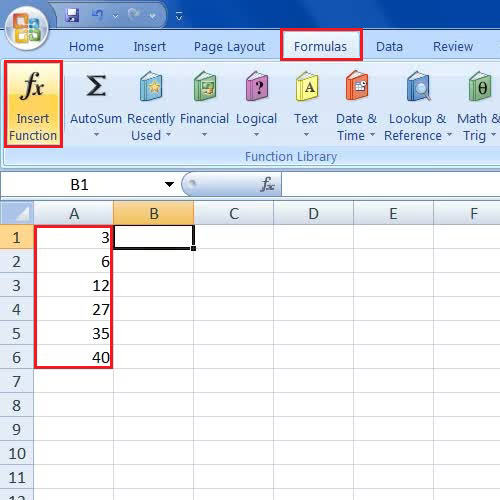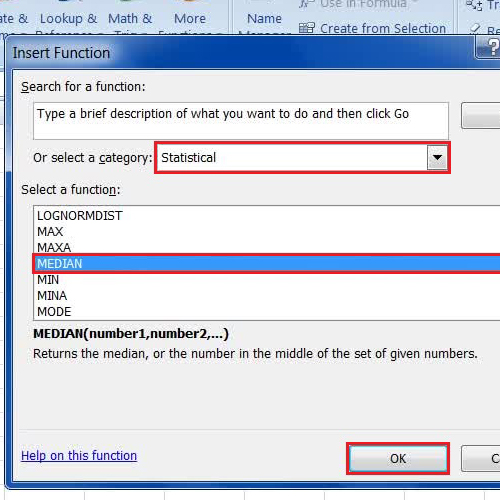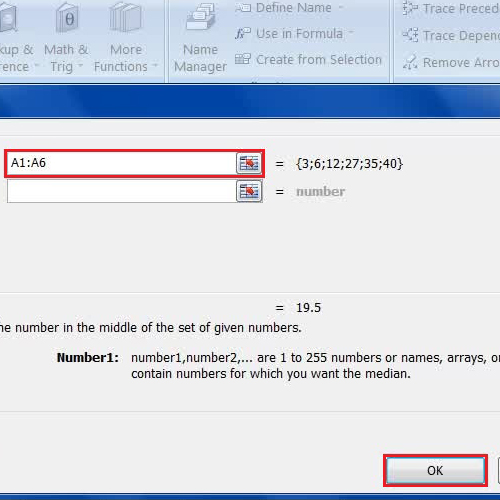## How to Use Median Function in Excel

A Median is a function which is used in statistics and probability theory. This function represents a value by separating the higher half of a list of numbers from the lower half. It is widely used in calculation of population related information and in other statistical data. In Excel, the median function provides its users to use this function in a very easy way. Here we will show you a simple way to apply a median function over a list of numbers to find out the value.

Step # 1 – Insert the data for Finding out the Median

In this tutorial, we will guide you on how to use median function in excel. First of all, open up a blank Excel sheet and insert few random numbers in a row. Now click on the next cell, select the “Formula” option from the menu and click on the “Insert Function” button.Step # 2 – Choose the Median Function

Once the Insert Function option has opened, choose the category as “Statistical”, select the Median function in Excel from the menu and click on the “OK” button to load it.Step # 3 – Select the Data from the Column

Now select and drag the row in which you have entered numbers to load them into the function and click on the “OK” button. Here you can see that the cell is showing the Median value of these numbers.

The Excel median function is easy to use and useful when analyzing statistical data.